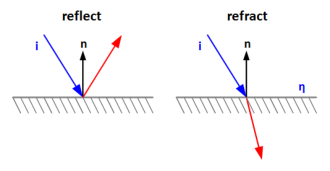# Reflect and Refract Functions

Uwaga! Informacje na tej stronie mają ponad 5 lat. Nadal je udostępniam, ale prawdopodobnie nie odzwierciedlają one mojej aktualnej wiedzy ani przekonań.

Mon
24
Aug 2009

Every game developer must have some knowledge about maths and especially geometry. Math library for game developers always contains structures like vector, matrix and functions like vector normalization or matrix inversion. But high level shader languages, designed to do geometrical computations, have many more interesting intrinsic functions. Some of more complex ones are reflect and refract, which calculate vector reflection and refraction in relation to the normal of a surface. How to implement them in C++?Reflection works according the simple rule that "angle of incidence equals angle of reflection". Although there are no angles in the formula - all the necessary work is done by dot product.

```void Reflect(VEC3 &out, const VEC3 &incidentVec, const VEC3 &normal)
{
out = incidentVec - 2.f * Dot(incidentVec, normal) * normal;
}```

For reflect function, the formula is given in th HLSL documentation, but for refract function it is not. It took me some time to find the implementation, but finally I've found it in the GLSL Specification (thanks KriS!). So here is the code converted to C++. Eta is the refraction index (e.g. 1.5f).

```inline void Refract(
VEC3 &out, const VEC3 &incidentVec, const VEC3 &normal, float eta)
{
float N_dot_I = Dot(normal, incidentVec);
float k = 1.f - eta * eta * (1.f - N_dot_I * N_dot_I);
if (k < 0.f)
out = VEC3(0.f, 0.f, 0.f);
else
out = eta * incidentVec - (eta * N_dot_I + sqrtf(k)) * normal;
}```

These functions are part of my library: CommonLib.